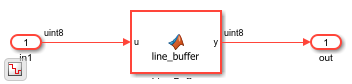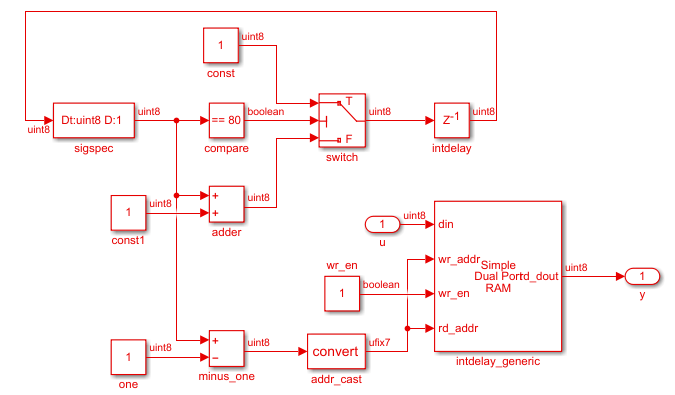# RAM Mapping with the MATLAB Function Block

This example shows how to map persistent arrays to RAM by using the `MapPersistentVarsToRAM` block-level parameter. The RAM size must be greater than or equal to the `RAMMappingThreshold`. The resource report shows the difference in area improvements resulting from RAM mapping.

### Line Buffer Model

Open the model `hdlcoder_ram_mapping_matlab_function`.

```open_system('hdlcoder_ram_mapping_matlab_function') set_param('hdlcoder_ram_mapping_matlab_function', 'SimulationCommand', 'Update') ```The DUT Subsystem in the model drives a `Line Buffer` MATLAB Function block.

```open_system('hdlcoder_ram_mapping_matlab_function/HDL_DUT') ```To see the MATLAB® code implementation of the line buffer, open the MATLAB Function block.

```open_system('hdlcoder_ram_mapping_matlab_function/HDL_DUT/Line Buffer') ```### Generate HDL Code

1. Enable generation of the resource utilization report. The report displays the number of adders, subtractors, multipliers, registers, and RAMs that the design consumes.

```hdlset_param('hdlcoder_ram_mapping_matlab_function', 'resourcereport', 'on') ```

2. Generate HDL code for the `HDL_DUT` Subsystem.

```makehdl('hdlcoder_ram_mapping_matlab_function/HDL_DUT') ```

HDL Coder™ displays the Code Generation Report. In the report, select the High-Level Resource Report section. The design consumes `81` registers and `648` 1-bit registers. By default, the `MapPersistentVarsToRAM` property is disabled and the code generator does not infer or consume RAM resources.### Enable RAM Mapping and Generate HDL Code

1. Enable the `MapPersistentVarsToRAM` HDL parameter on the MATLAB Function block.

```ml_subsys = 'hdlcoder_ram_mapping_matlab_function/HDL_DUT/Line Buffer'; hdlset_param(ml_subsys, 'MapPersistentVarsToRAM', 'on') ```

2. Generate HDL code for the `HDL_DUT` Subsystem.

```makehdl('hdlcoder_ram_mapping_matlab_function/HDL_DUT') ```

In the Code Generation Report, select the High-Level Resource Report section. The design consumes one register, eight 1-bit registers, and one RAM. The number of RAMs inferred depends on the `RAMMappingThreshold` that you specify. See RAM Mapping Parameters.### RAM Mapping with MATLAB Datapath Architecture

The `MATLAB Datapath` architecture treats the MATLAB Function block like a regular Subsystem. The architecture converts the MATLAB code that you wrote to a dataflow representation in Simulink®. HDL Coder can then more widely use optimizations across the MATLAB Function block with other Simulink blocks in your model.

1. Enable the `MATLAB Datapath` HDL architecture and then set the `MapPersistentVarsToRAM` parameter on the MATLAB Function block.

```hdlset_param(ml_subsys, 'Architecture', 'MATLAB Datapath') hdlset_param(ml_subsys, 'MapPersistentVarsToRAM', 'on') ```

2. Generate HDL code for the `HDL_DUT` Subsystem.

```makehdl('hdlcoder_ram_mapping_matlab_function/HDL_DUT') ```

The High-Level Resource Report indicates that the design consumes the same number of resources as the design that used the default architecture of the MATLAB Function block. To see how `MATLAB Datapath` architecture modifies the MATLAB code to a Simulink dataflow representation, open the generated model `gm_hdlcoder_ram_mapping_matlab_function` and navigate to the `HDL_DUT` Subsystem. There is a `Line Buffer` Subsystem in place of the MATLAB Function block. Inside the Subsystem block is the dataflow representation that displays a RAM block inferred.To learn about design patterns that enable efficient RAM mapping of persistent arrays in MATLAB Function blocks, see the `eml_hdl_design_patterns/RAMs` library.